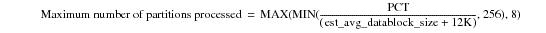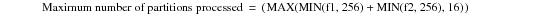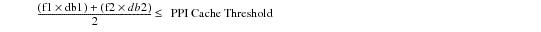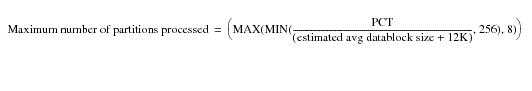# How Teradata Database Controls Memory Usage by Partitions - Teradata Database

Product
Release Number
15.10
Language
English (United States)
Last Update
2018-10-06
Product Category
Software

### How Teradata Database Controls Memory Usage by Partitions

The system associates each data block that contains a non‑eliminated partition with each context. Whenever possible, the set of data blocks containing the partitions being processed is kept in memory to improve its processing.

If enough memory is not available to contain all the relevant data blocks, some of those blocks must be swapped to disk. While a minimum amount of swapping is acceptable, excessive swapping degrades system performance. The function of the PPICacheThrP parameter is to control the amount of partitioning data block swapping.

PPICacheThrP does this by controlling the memory usage of partitioned operations. Larger values improve the performance of partitioned operations as long as the following restrictions are observed:

• Data blocks are kept memory‑resident.
• If they must be swapped to disk, performance might begin to degrade.

• The number of contexts does not exceed the number of populated, non‑eliminated partitions being processed.
• In this case, increasing the value of PPICachThrP does not improve performance because each partition has a context, and additional contexts would not be used.

Note that the maximum PPI cache that can be allocated is 100 MB regardless of the setting for PPICacheThrP.

Teradata Database uses the value of PCT for the following operations on a partitioned table.

• Joins
• Aggregation
• Merge spooling
• The equations described in the following bullets define the maximum number of partitions and contexts to process at a time based on the available PCT as determined from PPICacheThrP. If there are fewer populated non‑eliminated partitions, then the system partitions all table partitions simultaneously.

The following bullets describe the partitioning operations that use PCT.

• Joins on a partitioned table.
• If a join is performed on the primary index of partitioned table or spool and the other table or spool is not partitioned, the maximum number of partitions processed at one time from the partitioned table or spool is equal to the following calculation:If the join is made on the primary index of two partitioned relations, then the maximum number of partitions processed at a time from the relations is calculated as follows:where:For each partition that is being processed, one data block is memory‑resident. The definitions for the variables in this equation are as follows:

 Equation element … Specifies the … f1 number of partitions to be processed at one time from the left relation in the join as determined by the Optimizer. f2 number of partitions to be processed at one time from the right relation in the join as determined by the Optimizer. db1 estimated average datablock size of the left relation in the join. db2 estimated average datablock size of the right relation in the join.
• Aggregation of a partitioned table.
• If the system aggregates on the primary index of a partitioned table, the maximum number of partitions processed at one time from the table is calculated as follows:Note that for each partition that is being processed, one data block is memory‑resident.

You should decrease the value of PPICacheThrP if memory contention occurs during these types of operations.